# 1 Introduction

Rare Variant Sharing (RVS) implements tests of association and linkage between rare genetic variant genotypes and a dichotomous phenotype, e.g. a disease status, in family samples (Sherman et al. (2018)). The tests are based on probabilities of rare variant sharing by relatives under the null hypothesis of absence of linkage and association between the rare variants and the phenotype and apply to single variants or multiple variants in a region (e.g. gene-based test).

# 2 Setting up Pedigree Data

For this example experiment we will consider four family types. A pair of first cousins, a pair of second cousins, a triple of first cousins, and a triple of second cousins. RVS comes with several example pedigrees and these four types can be found in the samplePedigrees list.

data(samplePedigrees)

# store the pedigrees
fam_type_A <- samplePedigrees$firstCousinPair fam_type_B <- samplePedigrees$secondCousinPair
fam_type_C <- samplePedigrees$firstCousinTriple fam_type_D <- samplePedigrees$secondCousinTriple

# re-label the family ids for this example
fam_type_A$famid <- rep('SF_A', length(fam_type_A$id))
fam_type_B$famid <- rep('SF_B', length(fam_type_B$id))
fam_type_C$famid <- rep('SF_C', length(fam_type_C$id))
fam_type_D$famid <- rep('SF_D', length(fam_type_D$id))

## 2.2 Plotting a Pedigree

In order to see the pedigree structure we can use the plot function provided by the kinship2 package. In this family we have three second cousins that have been sequenced.

plot(fam_type_D)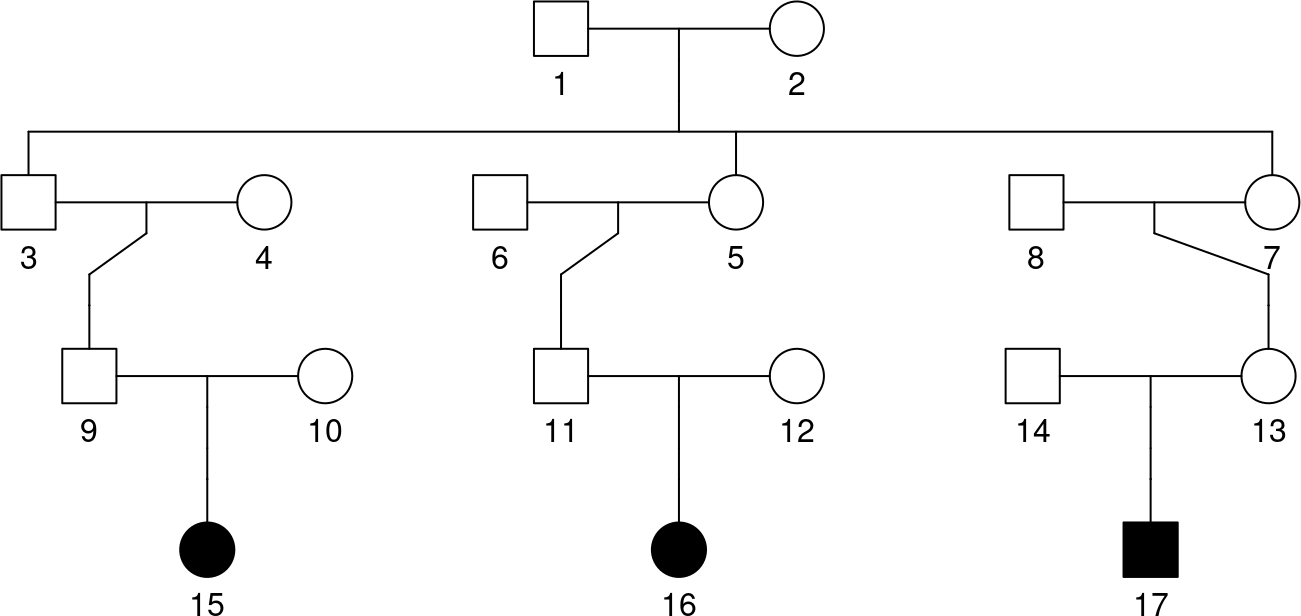# 3 Calculating Sharing Probabilities

## 3.1 Sharing Probability for One Family, One Variant

The simplest use of the RVS package is to compute the probability that all sequenced subjects in a pedigree share a rare variant, given that it is seen it at least one of the subjects. For more information about this calculation see the documentation for the function RVsharing and the Appendix.

In this case we compute the probability for the family of three second cousins. Note that in the case of a single family and variant, the sharing probability can be interpreted as a p-value.

p <- RVsharing(fam_type_D)
## Probability subjects 15 16 17 among 15 16 17 share a rare variant: 0.001342

## 3.2 P-Value for Multiple Families, One Variant

In the case of a single variant seen across multiple families, we can compute the individual sharing probabilities with RVsharing, but the sharing probabilities can no longer be interpreted as a p-value for the sharing pattern of the variant across the families. The function multipleFamilyPValue can be used to compute the p-value which is defined as the sum of all sharing probabilities across families at most as large as the sharing probability observed.

# compute the sharing probabilities for all families
fams <- list(fam_type_A, fam_type_B, fam_type_C, fam_type_D)
sharing_probs <- suppressMessages(RVsharing(fams))
signif(sharing_probs, 3)
##    SF_A    SF_B    SF_C    SF_D
## 0.06670 0.01590 0.01180 0.00134
# compute p-value for this sharing pattern
sharing_pattern <- c(TRUE, TRUE, FALSE, FALSE)
names(sharing_pattern) <- names(sharing_probs)
multipleFamilyPValue(sharing_probs, sharing_pattern)
##  0.002125543

The sharing_pattern vector indicates whether or not the variant is observed in all sequenced subjects.

## 3.3 P-Value for Multiple Families, Multiple Variants

The function multipleVariantPValue generalizes multipleFamilyPValue across multiple variants. This function takes a SnpMatrix instead of a specific sharing pattern. The behavior of this function could be achieved by converting every column of a SnpMatrix into a sharing pattern across families and applying multipleFamilyPValue across the columns.

The first step is reading in the genotype data. See the Data Input vignette in the snpStats package for examples using different file types. Here we use a pedigree file in the LINKAGE format. See here for an example of reading genotypes data from a Variant Call Format (VCF) file.

pedfile <- system.file("extdata/sample.ped.gz", package="RVS")
sample <- snpStats::read.pedfile(pedfile, snps=paste('variant', LETTERS[1:20], sep='_'))

In this data set we have 3 copies of each family type. The sharing probabilities for this set of families are:

A_fams <- lapply(1:3, function(i) samplePedigrees$firstCousinPair) B_fams <- lapply(1:3, function(i) samplePedigrees$secondCousinPair)
C_fams <- lapply(1:3, function(i) samplePedigrees$firstCousinTriple) D_fams <- lapply(1:3, function(i) samplePedigrees$secondCousinTriple)
fams <- c(A_fams, B_fams, C_fams, D_fams)
famids <- unique(sample$fam$pedigree)
for (i in 1:12)
{
fams[[i]]$famid <- rep(famids[i], length(fams[[i]]$id))
}
sharingProbs <- suppressMessages(RVsharing(fams))
signif(sharingProbs, 3)
##   SF_A1   SF_A2   SF_A3   SF_B1   SF_B2   SF_B3   SF_C1   SF_C2   SF_C3   SF_D1
## 0.06670 0.06670 0.06670 0.01590 0.01590 0.01590 0.01180 0.01180 0.01180 0.00134
##   SF_D2   SF_D3
## 0.00134 0.00134

When we call the function on the genotypes from a snpMatrix as follows, it converts them into a sharing pattern assuming the rare variant is the allele with the lowest frequency in the family sample:

result <- multipleVariantPValue(sample$genotypes, sample$fam, sharingProbs)
## Ignoring 0 variants not present in any subject
ppvals <- result$potential_pvalues ppvals_sub <- ppvals[names(pvals)] # subset potential p-values plot(-log10(ppvals[order(ppvals)]), ylab="-log10 p-value", col="blue", type="l", xaxt="n", xlab="variants", ylim=c(0,8)) xlabel <- sapply(names(ppvals)[order(ppvals)], function(str) substr(str, nchar(str), nchar(str))) axis(1, at=1:length(ppvals), labels=xlabel) points(-log10(pvals[order(ppvals_sub)]), pch=20, cex=1.3) bcut <- 0.05/(1:20) lines(1:20,-log10(bcut),col="red",type="b",pch=20)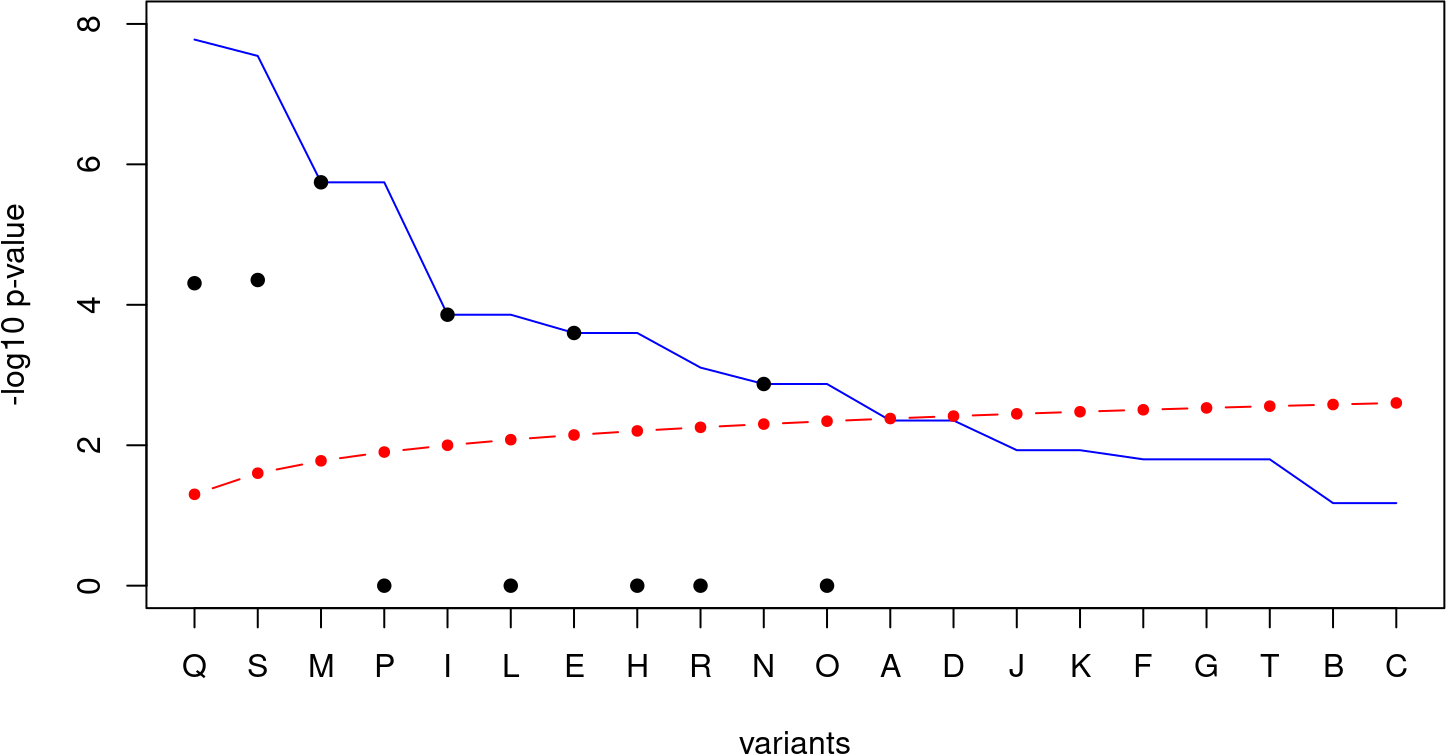## 3.5 Minor Allele Frequency Sensitivity Analysis When the minor allele frequency (MAF) is known in the population, then an exact sharing probability can be calculated using the alleleFreq parameter. Here we analyze the sensitivity of our p-values to the population MAF, using the 3 most significant variants. Note that variants which don’t reach their potential p-values, indicating some families only have partial sharing, are much more sensitive to the MAF. # calculate p-values for each MAF freq <- seq(0,0.05,0.005) variants <- names(sort(result$pvalues))[1:3]
pvals <- matrix(nrow=length(freq), ncol=length(variants))
pvals[1,] = sort(result$pvalues)[1:3] for (i in 2:length(freq)) { sharingProbs <- suppressMessages(RVsharing(fams, alleleFreq=freq[i])) pvals[i,] <- multipleVariantPValue(sample$genotypes[,variants], sample$fam, sharingProbs)$pvalues
}
## Ignoring 0 variants not present in any subject
## Ignoring 0 variants not present in any subject
## Ignoring 0 variants not present in any subject
## Ignoring 0 variants not present in any subject
## Ignoring 0 variants not present in any subject
## Ignoring 0 variants not present in any subject
## Ignoring 0 variants not present in any subject
## Ignoring 0 variants not present in any subject
## Ignoring 0 variants not present in any subject
## Ignoring 0 variants not present in any subject
colnames(pvals) <- variants

# plot p-values as a function of MAF
plot(NULL, xlim=c(min(freq),max(freq)), ylim=c(0,max(pvals)), type='l',
xlab="minor allele frequency", ylab="p-value",
main="sensitivity of p-value to allele frequency in three variants")
lines(freq, pvals[,1], col="black")
lines(freq, pvals[,2], col="red")
lines(freq, pvals[,3], col="blue")
legend(min(freq), max(pvals), legend=colnames(pvals), col=c("black", "red", "blue"), lty=1)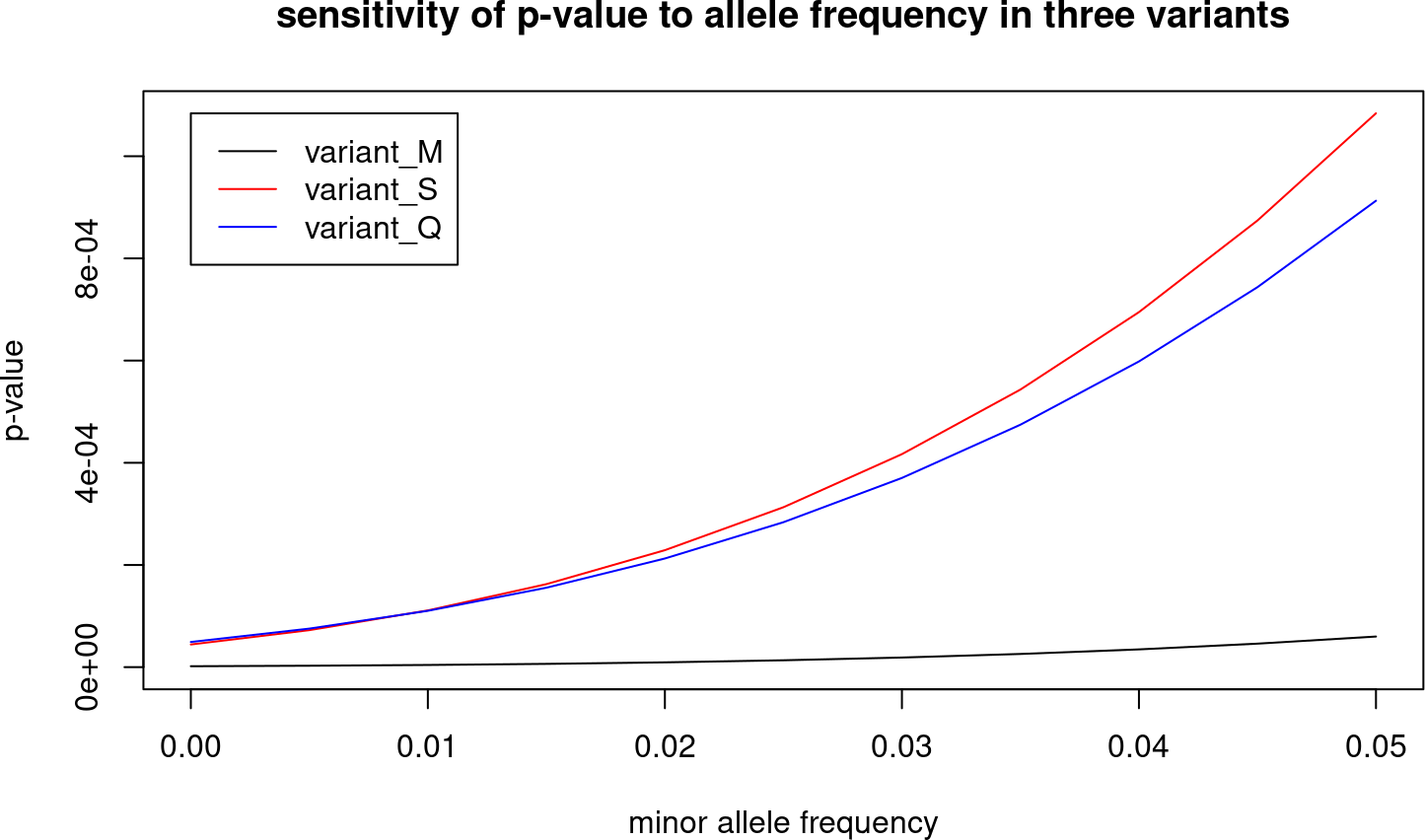# 4 Joint analysis of multiple variants

The power of single variant analyses is limited due to the small number of families where a rare variant is seen (often a single family). Even if no individual variant has a significant p-value, it is possible for multiple variants considered across multiple families to exhibit an unusual amount of sharing. The procedure to test multiple rare variants depends whether the variants are far apart or close together in a short genomic region, spanning a single gene for instance.

## 4.1 Enrichment Test

The enrichmentPValue function can compute a single p-value for all variants seen in all families assuming the variants are independent. This assumption is reasonable when variants are sufficiently far apart to be unlinked, such as rare deletions scattered over the whole genome as analyzed by Fu et al. (2017). The computation is implemented using a binary tree algorithm described by Fu et al. (2017). When calculating this p-value, note that a very small p-value may result in a very long computation time. Because of this, we can pass a minimum p-value threshold, where the greater of this threshold and the actual p-value will be returned.

enrichmentPValue(sample$genotypes, sample$fam, sharingProbs, 0.001)
## Ignoring 0 variants not present in any subject
##  0.001

## 4.2 Gene-based analysis

Joint analysis of rare variants within a gene (typically single nucleotide variants and short indels, possibly filtered based on functional annotations) is another approach to increase statistical power. Here the assumption of independence of rare variants does not hold when variants are seen in the same family, and the solution described by Bureau et al. (2018) and implemented in the RVgene function is to keep the variant with the sharing pattern having the lowest probability (usually the variant shared by the largest number of affected relatives in the family). The gene-based analysis with the RVgene function is illustrated in section 4.2 along with another new feature: the partial sharing test.

# 5 Partial sharing test

Phenocopies, diagnosis error and intra-familial genetic heterogeneity in complex disorders result in disease susceptibility variants being shared by a subset of affected subjects. In order to detect such causal variants, a partial sharing test was defined by Bureau et al. (2018) where the p-value is the probability of sharing events as or more extreme as the observed event. A more extreme sharing event is defined as having lower probability and involving more carriers of the variant.

## 5.1 Precomputing Sharing Probabilities and Number of Carriers for all Possible Carrier Subsets

In order to perfore the partial sharing test, the RVgene function requires the lists pattern.prob.list of vectors of sharing probabilities and N.list of number of carriers for all possible affected carrier subsets in each family in the sample being analyzed. The arguments of the RVsharing function allowing the computation of sharing probabilities by a subset of affected subjects are described here. The elements of both of these lists must have the same names as the pedigree objects in the ped.listfams argument. When all affected subjecs in a family are final descendants, the sharing probabilities and number of carriers for all subsets can be generated automatically. Here is an exanple with three second cousins:

carriers = c(15,16,17)
carrier.sets = list()
for (i in length(carriers):1)
carrier.sets = c(carrier.sets, combn(carriers,i,simplify=FALSE))
fam15157.pattern.prob = sapply(carrier.sets,function (vec) RVsharing(samplePedigrees$secondCousinTriple,carriers=vec)) ## Probability subjects 15 16 17 among 15 16 17 share a rare variant: 0.001342 ## Probability subjects 15 16 among 15 16 17 share a rare variant: 0.009396 ## Probability subjects 15 17 among 15 17 16 share a rare variant: 0.009396 ## Probability subjects 16 17 among 16 17 15 share a rare variant: 0.009396 ## Probability subjects 15 among 15 16 17 share a rare variant: 0.3235 ## Probability subjects 16 among 16 15 17 share a rare variant: 0.3235 ## Probability subjects 17 among 17 15 16 share a rare variant: 0.3235 fam15157.N = sapply(carrier.sets,length) When the splitPed option is TRUE, the generation of all carrier subsets is performed within the RVsharing function, which then returns the vector of sharing probabilities for all subsets. So the following code is equivalent to the sapply of RVsharing above: fam15157.pattern.prob = RVsharing(samplePedigrees$secondCousinTriple,splitPed=TRUE)
## Founder  1  subped size  17
## Founder  4  subped size  5
## Founder  6  subped size  5
## Founder  8  subped size  5
## Founder  10  subped size  3
## Founder  12  subped size  3
## Founder  14  subped size  3
## Probability every subset of subjects among 15 16 17 share a rare variant:
##  0.001342 0.009396 0.009396 0.009396 0.323500 0.323500 0.323500

While this code applies to any configuration of affected final descendants, symmetries in the relationships of these third cousins results in equal sharing probabilities for multiple subsets. Subsets with the same probabilities are equivalent, and the optional argument nequiv.list can be used to indicate the number of equivalent subset for each sharing probability. While shorter vectors in pattern.prob.list and N.list result in more efficient computation, identification of the equivalent subsets is not easily automated, and will usually require custom code for each pedigree in a sample. With three second cousins we can use:

fam15157.pattern.prob = c(RVsharing(samplePedigrees$secondCousinTriple,carriers=c(15,16,17)), RVsharing(samplePedigrees$secondCousinTriple,carriers=c(15,16)),
RVsharing(samplePedigrees$secondCousinTriple,carriers=c(15))) ## Probability subjects 15 16 17 among 15 16 17 share a rare variant: 0.001342 ## Probability subjects 15 16 among 15 16 17 share a rare variant: 0.009396 ## Probability subjects 15 among 15 16 17 share a rare variant: 0.3235 fam15157.N = 3:1 fam15157.nequiv = c(1,3,3) It is then easy to check that the distribution sums to 1: sum(fam15157.pattern.prob*fam15157.nequiv) ##  1 When some affected subjects are not final descendants, some subsets are incompatible with a variant being IBD among carriers. Assume individual 3, the grand-father of subject 15 in family 15157, is also affected and his genotype is available. fam15157 <- samplePedigrees$secondCousinTriple
fam15157$affected = 1 plot(fam15157)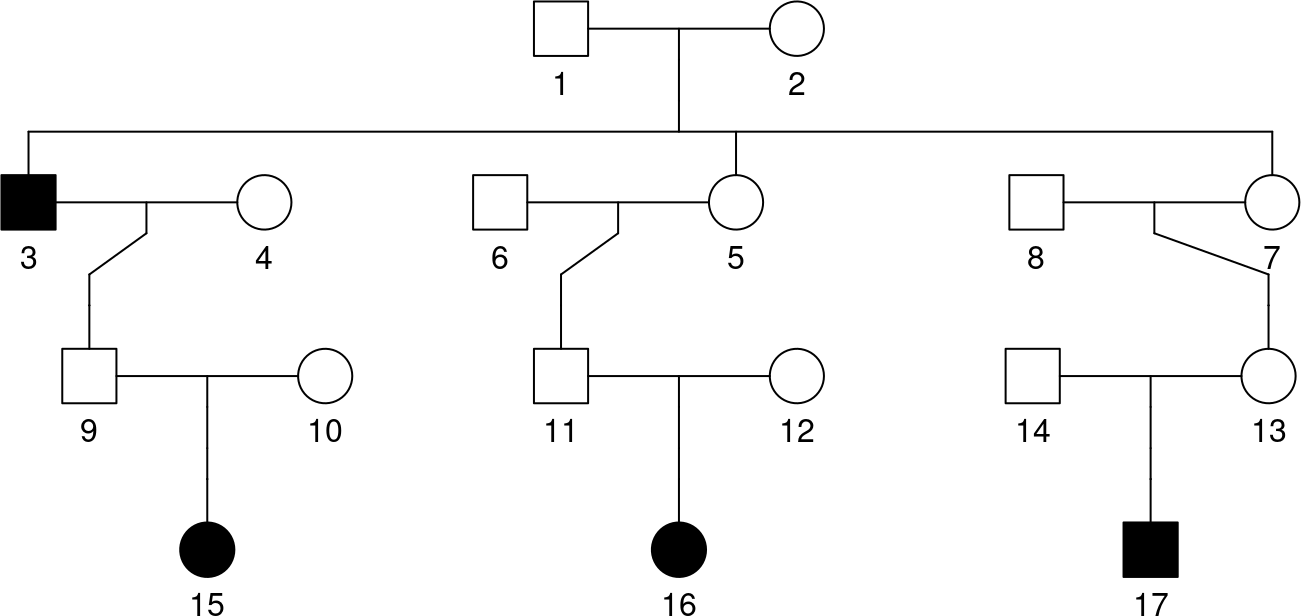Then the carrier subsets (15,16,17), (15,16) and (15,17) involving subject 15 but not 3 are incompatible with sharing IBD and must be removed from the list of subsets. The code then becomes: carriers = c(3,15,16,17) carrier.sets = list() for (i in length(carriers):1) carrier.sets = c(carrier.sets, combn(carriers,i,simplify=FALSE)) carrier.sets ## [] ##  3 15 16 17 ## ## [] ##  3 15 16 ## ## [] ##  3 15 17 ## ## [] ##  3 16 17 ## ## [] ##  15 16 17 ## ## [] ##  3 15 ## ## [] ##  3 16 ## ## [] ##  3 17 ## ## [] ##  15 16 ## ## [] ##  15 17 ## ## [] ##  16 17 ## ## [] ##  3 ## ## [] ##  15 ## ## [] ##  16 ## ## [] ##  17 carrier.sets = carrier.sets[-c(5,9,10)] fam15157.pattern.prob = sapply(carrier.sets,function (vec) RVsharing(fam15157,carriers=vec,useAffected=TRUE)) ## Probability subjects 3 15 16 17 among 3 15 16 17 share a rare variant: 0.001121 ## Probability subjects 3 15 16 among 3 15 16 17 share a rare variant: 0.007848 ## Probability subjects 3 15 17 among 3 15 16 17 share a rare variant: 0.007848 ## Probability subjects 3 16 17 among 3 15 16 17 share a rare variant: 0.003363 ## Probability subjects 3 15 among 3 15 16 17 share a rare variant: 0.05493 ## Probability subjects 3 16 among 3 15 16 17 share a rare variant: 0.02354 ## Probability subjects 3 17 among 3 15 16 17 share a rare variant: 0.02354 ## Probability subjects 16 17 among 3 15 16 17 share a rare variant: 0.004484 ## Probability subjects 3 among 3 15 16 17 share a rare variant: 0.1648 ## Probability subjects 15 among 3 15 16 17 share a rare variant: 0.2152 ## Probability subjects 16 among 3 15 16 17 share a rare variant: 0.2466 ## Probability subjects 17 among 3 15 16 17 share a rare variant: 0.2466 fam15157.N = sapply(carrier.sets,length) Notice the use of the option useAffected=TRUE with affected subjects who are not final descendants. Again, one can check that the distribution sums to 1: sum(fam15157.pattern.prob) ##  1 Precomputed sharing probabilities and numbers of carriers can be used directly to obtain p-values of observed sharing events, by summing the probability of all events as or more extreme as the one observed (both in terms of sharing probability and number of carriers), i.e. this is a one-sided exact test. For instance, if subjects 3, 16 and 17 share a rare variant, the probability of that event is pobs = RVsharing(fam15157,carriers=c(3,16,17),useAffected=TRUE) ## Probability subjects 3 16 17 among 3 15 16 17 share a rare variant: 0.003363 The p-value of that sharing event is then: sum(fam15157.pattern.prob[fam15157.pattern.prob<=pobs & fam15157.N >= 3]) ##  0.004484305 The RVgene function enables these computations with more than one family harboring the same or different variants. Once the vectors of sharing probabilities and number of carriers have been computed for all families in the sample, they need to be stored in lists. We return to the original second cousin triple family and add a first and second cousin triple family. Then we create the lists of pattern probabilities, number of equivalent subsets and number of carriers in the subsets. fam15157.pattern.prob = c(RVsharing(samplePedigrees$secondCousinTriple,carriers=c(15,16,17)),
RVsharing(samplePedigrees$secondCousinTriple,carriers=c(15,16)), RVsharing(samplePedigrees$secondCousinTriple,carriers=c(15)))
## Probability subjects 15 16 17 among 15 16 17 share a rare variant: 0.001342
## Probability subjects 15 16 among 15 16 17 share a rare variant: 0.009396
## Probability subjects 15 among 15 16 17 share a rare variant: 0.3235
fam15157.N = 3:1
fam15157.nequiv = c(1,3,3)

fam28003.pattern.prob = c(RVsharing(samplePedigrees$firstAndSecondCousinsTriple,carriers=c(36,104,110)), RVsharing(samplePedigrees$firstAndSecondCousinsTriple,carriers=c(36,104)),
RVsharing(samplePedigrees$firstAndSecondCousinsTriple,carriers=c(104,110)), RVsharing(samplePedigrees$firstAndSecondCousinsTriple,carriers=c(36)),
RVsharing(samplePedigrees$firstAndSecondCousinsTriple,carriers=c(104))) ## Probability subjects 36 104 110 among 36 104 110 share a rare variant: 0.00277 ## Probability subjects 36 104 among 36 104 110 share a rare variant: 0.00831 ## Probability subjects 104 110 among 104 110 36 share a rare variant: 0.04155 ## Probability subjects 36 among 36 104 110 share a rare variant: 0.3352 ## Probability subjects 104 among 104 36 110 share a rare variant: 0.3019 fam28003.N = c(3,2,2,1,1) fam28003.nequiv = c(1,2,1,1,2) ex.pattern.prob.list = list("15157"=fam15157.pattern.prob,"28003"=fam28003.pattern.prob) ex.nequiv.list = list("15157"=fam15157.nequiv,"28003"=fam28003.nequiv) ex.N.list = list("15157"=fam15157.N,"28003"=fam28003.N) ## 5.2 Example of Analysis of the Rare Variants in the Genomic Sequence of a Gene We now turn to the analysis of variants observed in the simulated genomic sequence of the gene PEAR1 in a sample of related affected subjects. The processing of the sequence data results in Variant Call Format (VCF) files, which can be read into R with the function readVcf from the variantAnnotation package. Two VCF objects obtained with readVcf from VCF files of sequence data for the second cousin triple and first and second cousin triple families are contained in the famVCF data. These VCF files are converted to snpMatrix objects using the genotypeToSnpMatrix function. data(famVCF) fam15157.snp = VariantAnnotation::genotypeToSnpMatrix(fam15157.vcf) ## Warning: replacing previous import 'utils::findMatches' by ## 'S4Vectors::findMatches' when loading 'AnnotationDbi' ## non-single nucleotide variations are set to NA ## Warning in .local(x, ...): non-diploid variants are set to NA fam28003.snp = VariantAnnotation::genotypeToSnpMatrix(fam28003.vcf) ## non-single nucleotide variations are set to NA ## Warning in .local(x, ...): non-diploid variants are set to NA RVgene requires lists of the snpMatrix and pedigree objects for these two families. The names given to the elements of these lists are not used by RVgene and are thus arbitrary. Family IDs are extracted from the famid element of the pedigree objects. Please note that currently RVgene does not accept a pedigreeList, but only a plain list of pedigree objects. ex.SnpMatrix.list = list(fam15157=fam15157.snp$genotypes,fam28003=fam28003.snp$genotypes) ex.ped.obj = list(fam15157=samplePedigrees$secondCousinTriple,fam28003=samplePedigrees$firstAndSecondCousinsTriple) In the sequence segment, one can specify which variants are rare and possibly satisfy other filtering criteria (e.g. coding variants) using the sites argument. Here, we will focus on two sites: 92 where the three second cousins of family 15157 share the rare allele and 119 where the two first cousins of family 28003 share the rare allele but not their second cousin. sites = c(92,119) ex.SnpMatrix.list[["fam15157"]][,sites]@.Data ## rs187756653 ## 1 00 ## 2 00 ## 3 00 ## 4 00 ## 5 00 ## 6 00 ## 7 00 ## 8 00 ## 9 00 ## 10 00 ## 11 00 ## 12 00 ## 13 00 ## 14 00 ## 15 02 ## 16 02 ## 17 02 ex.SnpMatrix.list[["fam28003"]][,sites]@.Data ## rs199628333 ## 3 00 ## 4 00 ## 6 00 ## 7 00 ## 11 00 ## 12 00 ## 13 00 ## 15 00 ## 27 00 ## 28 00 ## 36 01 ## 103 00 ## 104 02 ## 109 00 ## 110 02 Finally, the call to RVgene returns the P-value of the exact rare variant sharing test allowing for sharing by a subset of affected subjects (p), the P-value of the exact rare variant sharing test requiring sharing by all affected subjects (pall) and the minimum achievable p-value if all affected subjects were carriers of a rare variant (potentialp). RVgene(ex.SnpMatrix.list,ex.ped.obj,sites,pattern.prob.list=ex.pattern.prob.list,nequiv.list=ex.nequiv.list,N.list=ex.N.list,type="count") ##$p
##  0.000159884
##
## $pall ##  0.001342282 ## ##$potentialp
##  3.718232e-06

# 6 Appendix

## 6.1 Rare Variant Sharing Probability Assuming One Founder Introduces the Variant

In this case, we assume the variant is rare enough so that the probability of more than one founder introducing it to the pedigree is negligible. This is the default scenario for RVsharing.

We define the following random variables:

*$$C_i$$: Number of copies of the RV received by subject $$i$$,

*$$F_j$$: Indicator variable that founder $$j$$ introduced one copy of the RV into the pedigree,

For a set of $$n$$ subjects descendants of $$n_f$$ founders we want to compute the probability $\begin{eqnarray*} P[\mbox{RV shared}] &=& P[C_1 = \dots = C_n = 1 | C_1 + \dots + C_n \geq 1] \nonumber \\[0.5em] &=& \frac{P[C_1 = \dots = C_n = 1 ]}{P[C_1 + \dots + C_n \geq 1]} \nonumber \\[0.5em] &=& \frac{\sum_{j=1}^{n_f} P[C_1 = \dots = C_n = 1 | F_j] P[F_j]} {\sum_{j=1}^{n_f} P[C_1 + \dots + C_n \geq 1 | F_j]P[F_j]}, \label{sharingp} \end{eqnarray*}$ where the expression on the third line results from our assumption of a single copy of that RV among all alleles present in the $$n_f$$ founders. The probabilities $$P[F_j] = {1 \over n_f}$$ cancel from the numerator and denominator.

## 6.2 Rare Variant Sharing Probabilities for a Subset of Affected Pedigree Members

By default, RVsharing will compute the probability that all of the final descendants share the variant given that it is seen in at least one of them. Final descendants are defined as subjects of the pedigree with no children. This event can be customized with the carriers and useAffected arguments.

If the argument carriers is provided, then the probability of all carriers having the variant given it is seen in at least one subject in the union of the final descendants and the carriers will be computed.

If the argument useAffected is TRUE and the pedigree has a slot for affected, then the probability of all carriers having the variant given it is seen in at least one affected will be computed.

These two arguments can be used individually or in combination, the only restriction is that carriers must be a subset of affected.

ped <- samplePedigrees$firstCousinTriple ped$affected <- 0
plot(ped)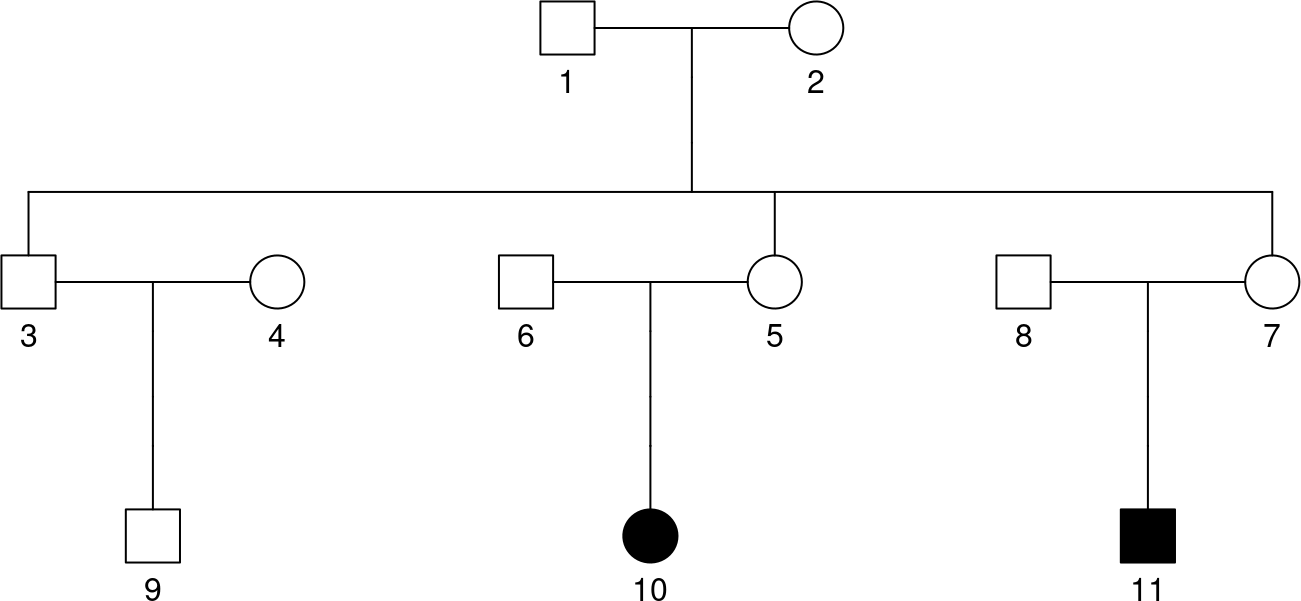p <- RVsharing(ped)
## Probability subjects 9 10 11 among 9 10 11 share a rare variant: 0.01176
p <- RVsharing(ped, useAffected=TRUE)
## Probability subjects 10 11 among 10 11 share a rare variant: 0.06667
p <- RVsharing(ped, carriers=c(7,9,10))
## Probability subjects 7 9 10 among 7 9 10 11 share a rare variant: 0.01064
p <- RVsharing(ped, carriers=c(10,11), useAffected=TRUE)
## Probability subjects 10 11 among 10 11 share a rare variant: 0.06667

## 6.3 Using Monte Carlo Simulation

RVsharing also allows for estimating sharing probabilities through Monte Carlo simulation. The primary use of this feature is for calculating sharing probabilities under non standard assumptions about the founders. However, this feature is available for the standard assumptions as well. To run a monte carlo simulation, specify all parameters as normal and additionally provide the nSim parameter specifying how many simulations should be run.

p <- RVsharing(samplePedigrees$firstCousinPair, alleleFreq=0.01) ## Probability subjects 7 8 among 7 8 share a rare variant: 0.07631 p <- RVsharing(samplePedigrees$firstCousinPair, alleleFreq=0.01, nSim=1e5)
## Probability subjects 7 8 among 7 8 share a rare variant: 0.07509

This method allows for more complex relationships among the founders to be given. RVsharing allows for a complete distribution among the founders to be passed in as the parameter founderDist. This function should accept a single argument, N, and should return a vector of length N with values in {0,1,2} representing the number of copies of the variant each founder has.

# assumption that 1 founder introduces variant
fDist <- function(N) sample(c(rep(0,N-1), 1))
p <- RVsharing(samplePedigrees$firstCousinPair, nSim=1e5, founderDist=fDist) ## Probability subjects 7 8 among 7 8 share a rare variant: 0.06659 p <- RVsharing(samplePedigrees$firstCousinPair)
## Probability subjects 7 8 among 7 8 share a rare variant: 0.06667